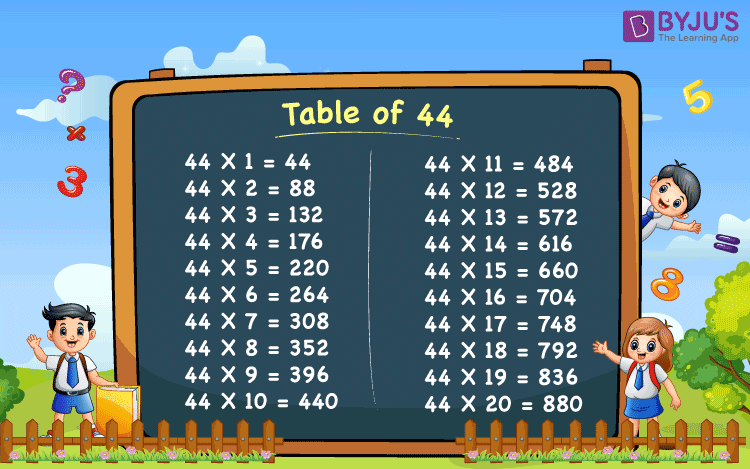# Table of 44

Table of 44 is a Maths multiplication table for the number forty-four – 44. This table helps in solving many simplification problems in maths. The students who are preparing for competitive exams can refer to these Tables from 1 to 100 to solve the numerical problems quickly so that they can save time. Here, you will get the downloadable PDF and chart of the multiplication table of 44.

## Table of 44 ChartWhat is the table of 44?

The table of 44 can be defined using the repeated addition of 44. For example, 44 times 6 can be calculated as:

44 × 6 = 44 + 44 + 44 + 44 + 44 + 44 = 264

Therefore, 44 × 6 = 264

Similarly, all the multiples of 44 can be obtained. The below table contains the multiplication of forty-four from 1 to 20.

## Multiplication Table of 44

 44 × 1 = 44 44 × 2 = 88 44 × 3 = 132 44 × 4 = 176 44 × 5 = 220 44 × 6 = 264 44 × 7 = 308 44 × 8 = 352 44 × 9 = 396 44 × 10 = 440 44 × 11 = 484 44 × 12 = 528 44 × 13 = 572 44 × 14 = 616 44 × 15 = 660 44 × 16 = 704 44 × 17 = 748 44 × 18 = 792 44 × 19 = 836 44 × 20 = 880

### How to Read Table of 44

The below sentences help in reading the forty-four times table from 1 to 10.

• One time forty-four is forty four
• Two times forty-four is eighty eight
• Three times forty-four is one hundred and thirty two
• Four times forty-four is one hundred and seventy six
• Five times forty-four is two hundred and twenty
• Six times forty-four is two hundred and sixty four
• Seven times forty-four is three hundred and eight
• Eight times forty-four is three hundred and fifty two
• Nine times forty-four is three hundred and ninety six
• Ten times forty-four is four hundred and forty

### Get More Maths Tables

To learn more multiplication tables, visit www.byjus.com and download BYJU’S – The Learning App for interactive and engaging videos.